# The Four Types of Estimable Functions

### Type II SS and Estimable Functions

Subsections:

For main-effects models and regression models, the general form of estimable functions can be manipulated to provide tests of hypotheses involving only the parameters of the effect in question. The same result can also be obtained by entering each effect in turn as the last effect in the model and obtaining the Type I SS for that effect. These are the Type II SS. Using a modified reversible sweep operator, it is possible to obtain the Type II SS without actually refitting the model.

Thus, the Type II SS correspond to the R notation in which each effect is adjusted for all other appropriate effects. For a regression model such as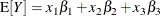the Type II SS correspond to

Effect

Type II SS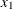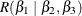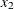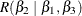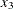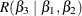For a main-effects model (A, B, and C as classification variables), the Type II SS correspond to

Effect

Type II SS

A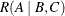B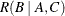C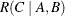As the discussion in the section A Three-Factor Main-Effects Model indicates, for regression and main-effects models the Type II SS provide an MRH for each effect that does not involve the parameters of the other effects.

In order to see what effects are appropriate to adjust for in computing Type II estimable functions, note that for models involving interactions and nested effects, in the absence of a priori parametric restrictions, it is not possible to obtain a test of a hypothesis for a main effect free of parameters of higher-level interactions effects with which the main effect is involved. It is reasonable to assume, then, that any test of a hypothesis concerning an effect should involve the parameters of that effect and only those other parameters with which that effect is involved. The concept of effect containment helps to define this involvement.

#### Contained Effect

Given two effects F1 and F2, F1 is said to be contained in F2 provided that the following two conditions are met:

• Both effects involve the same continuous variables (if any).

• F2 has more CLASS variables than F1 does, and if F1 has CLASS variables, they all appear in F2.

Note that the intercept effectis contained in all pure CLASS effects, but it is not contained in any effect involving a continuous variable. No effect is contained by.

Type II, Type III, and Type IV estimable functions rely on this definition, and they all have one thing in common: the estimable functions involving an effect F1 also involve the parameters of all effects that contain F1, and they do not involve the parameters of effects that do not contain F1 (other than F1).

#### Hypothesis Matrix for Type II Estimable Functions

The Type II estimable functions for an effect F1 have an
(before reduction to full row rank) of the following form:

• All columns ofassociated with effects not containing F1 (except F1) are zero.

• The submatrix ofassociated with effect F1 is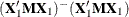.

• Each of the remaining submatrices ofassociated with an effect F2 that contains F1 is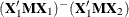.

In these submatrices,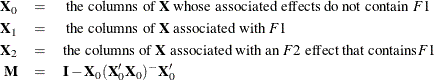For the model

class A B;
model Y = A B A*B;


the Type II SS correspond to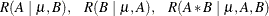for effects A, B, and A * B, respectively. For the model

class A B C;
model Y =  A B(A) C(A B);


the Type II SS correspond to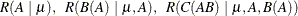for effects A,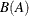and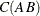, respectively. For the model

model Y = x x*x;


the Type II SS correspond to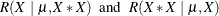for x and, respectively.

Note that, as in the situation for Type I tests, PROC MIXED and PROC GLIMMIX compute Type I hypotheses by sweeping, but their test statistics are not necessarily equivalent to the results of sequentially fitting with those procedures models that contain successively more effects; while PROC TRANSREG computes tests labeled as being Type II by leaving out each effect in turn, but the specific linear hypotheses associated with these tests might not be precisely the same as the ones derived from successively sweeping.

#### Example of Type II Estimable Functions

For afactorial with w observations per cell, the general form of estimable functions is shown in Table 15.5. Any nonzero values for L2, L4, and L6 can be used to constructvectors for computing the Type II SS for A, B, and A * B, respectively.

Table 15.5: General Form of Estimable Functions forFactorial

Effect

CoefficientL1

A1

L2

A2

L1L2

B1

L4

B2

L1L4

AB11

L6

AB12

L2L6

AB21

L4L6

AB22

L1L2L4 + L6

For a balancedfactorial with the same number of observations in every cell, the Type II estimable functions are shown in Table 15.6.

Table 15.6: Type II Estimable Functions for BalancedFactorial

Coefficients for Effect

Effect

A

B

A * B0

0

0

A1

L2

0

0

A2

L2

0

0

B1

0

L4

0

B2

0

L4

0

AB11

0.5L2

0.5L4

L6

AB12

0.5L2

–0.5L4

L6

AB21

–0.5L2

0.5L4

L6

AB22

–0.5L2

–0.5L4

L6

Now consider an unbalancedfactorial with two observations in every cell except the AB22 cell, which contains only one observation. The general form of estimable functions is the same as if it were balanced, since the same effects are still estimable. However, the Type II estimable functions for A and B are not the same as they were for the balanced design. The Type II estimable functions for this unbalancedfactorial are shown in Table 15.7.

Table 15.7: Type II Estimable Functions for UnbalancedFactorial

Coefficients for Effect

Effect

A

B

A * B0

0

0

A1

L2

0

0

A2

L2

0

0

B1

0

L4

0

B2

0

L4

0

AB11

0.6L2

0.6L4

L6

AB12

0.4L2

–0.6L4

L6

AB21

–0.6L2

0.4L4

L6

AB22

–0.4L2

–0.4L4

L6

By comparing the hypothesis being tested in the balanced case to the hypothesis being tested in the unbalanced case for effects A and B, you can note that the Type II hypotheses for A and B are dependent on the cell frequencies in the design. For unbalanced designs in which the cell frequencies are not proportional to the background population, the Type II hypotheses for effects that are contained in other effects are of questionable value.

However, if an effect is not contained in any other effect, the Type II hypothesis for that effect is an MRH that does not involve any parameters except those associated with the effect in question.

Thus, Type II SS are appropriate for the following models:

• any balanced model

• any main-effects model

• any pure regression model

• an effect not contained in any other effect (regardless of the model)

In addition to the preceding models, Type II SS are generally accepted by most statisticians for purely nested models.